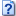Amazon Machine Learning – Take Two

Execution of Test

I performed the steps previously done using the new setup above. Without surprise the calculated AUC value returned was 1.0. For comparison I kept the score target to the default of 0.5.

I aggregated the results in this Excel sheet, which I have attached you for your reference.AML-take2.xlsx (62.1 KiB, 198 hits)

Results

Querying all 343 possible tuples, the model returned

• 335 correct predictions
• and 8 wrong predictions, which makes a 97.6% success rate or a 2.3% error rate.
• The wrong predictions are
• a = 2, b = 7, c = 3 (intermediate = 11, binary = true, prediction = false with a score of 418922)
• a = 3, b = 4, c = 2 (intermediate = 10, binary = false, prediction = true with a score of 618853)
• a = 3, b = 5, c = 5 (intermediate = 10, binary = false, prediction = true with a score of 543858)
• a = 4, b = 3, c = 2 (intermediate = 10, binary = false, prediction = true with a score of 808096)
• a = 4, b = 4, c = 6 (intermediate = 10, binary = false, prediction = true with a score of 696756)
• a = 4, b = 4, c = 7 (intermediate = 9, binary = false, prediction = true with a score of 534369)
• a = 5, b = 3, c = 5 (intermediate = 10, binary = false, prediction = true with a score of 529201)
• a = 7, b = 2, c = 4 (intermediate = 10, binary = false, prediction = true with a score of 55007)

It is noteworthy that out of the eight wrong predictions, seven of them is data which had been available to the training phase – and one of the four values is part of the test tuples (a = 7, b = 2, c = 4). Vice versa, this means that three out of four of our test tuples have been “guessed” correctly.

Also looking at the score of the false predictions there is a pattern to observe: Whilst the scores are very high for tuples which the model had seen during training (between 418,922 and 808,096 – the maximal score value in the entire data set is 999,999.9), the tuple which was not observed yet has a lower “confidence level” by a factor of 10.

Finally, looking at the intermediate values affected, you observe that only tuples with the intermediate values 9, 10 and 11 are subject to wrong predictions.

(continued on next page)

VN:F [1.9.22_1171]